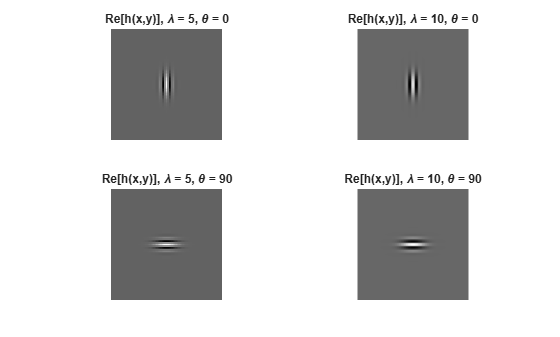Documentation

This is machine translation

Mouseover text to see original. Click the button below to return to the English version of the page.

gabor

Create Gabor filter or Gabor filter bank

Syntax

g = gabor(wavelength,orientation)
g = gabor(___,Name,Value,...)

Description

example

g = gabor(wavelength,orientation) creates a Gabor filter with the specified wavelength (in pixels/cycle) and orientation (in degrees). If you specify wavelength or orientation as vectors, gabor returns an array of gabor objects, called a filter bank, that contain all the unique combinations of wavelength and orientation. For example, if wavelength is a vector of length 2 and orientation is a vector of length 3, then the output array g is a vector of length 6. To apply the Gabor filters to an image, use the imgaborfilt function.
g = gabor(___,Name,Value,...) creates an array of Gabor filters using name-value pairs to control aspects of Gabor filter design. If you specify a vector of values, the output array g contains all the unique combinations of the input values.

Examples

collapse all

Create a sample image of a checkerboard.

A = checkerboard(20);

Create an array of Gabor filters.

wavelength = 20; orientation = [0 45 90 135]; g = gabor(wavelength,orientation);

Apply the filters to the checkerboard image.

outMag = imgaborfilt(A,g);

Display the results.

outSize = size(outMag); outMag = reshape(outMag,[outSize(1:2),1,outSize(3)]); figure, montage(outMag,'DisplayRange',[]); title('Montage of gabor magnitude output images.');Create array of Gabor filters.

g = gabor([5 10],[0 90]);

Visualize the real part of the spatial convolution kernel of each Gabor filter in the array.

figure; subplot(2,2,1) for p = 1:length(g) subplot(2,2,p); imshow(real(g(p).SpatialKernel),[]); lambda = g(p).Wavelength; theta = g(p).Orientation; title(sprintf('Re[h(x,y)], \\lambda = %d, \\theta = %d',lambda,theta)); endInput Arguments

collapse all

Wavelength of sinusoid, specified as a numeric scalar or vector, in pixels/cycle.

Data Types: single | double | int8 | int16 | int32 | int64 | uint8 | uint16 | uint32 | uint64

Orientation of filter in degrees, specified as a numeric scalar in the range [0 180], where the orientation is defined as the normal direction to the sinusoidal plane wave.

Data Types: single | double | int8 | int16 | int32 | int64 | uint8 | uint16 | uint32 | uint64

Name-Value Pair Arguments

Specify optional comma-separated pairs of Name,Value arguments. Name is the argument name and Value is the corresponding value. Name must appear inside quotes. You can specify several name and value pair arguments in any order as Name1,Value1,...,NameN,ValueN.

Example: g = gabor(4,90,'SpatialFrequencyBandwidth',1.5);

A numeric vector that defines the spatial-frequency bandwidth in units of Octaves. The spatial-frequency bandwidth determines the cutoff of the filter response as frequency content in the input image varies from the preferred frequency, 1/lambda. Typical values for spatial-frequency bandwidth are in the range [0.5 2.5].

Data Types: single | double | int8 | int16 | int32 | int64 | uint8 | uint16 | uint32 | uint64

Aspect ratio of Gaussian in spatial domain, specified as a numeric vector that defines the ratio of the semi-major and semi-minor axes of the Gaussian envelope: semi-minor/semi-major. This parameter controls the ellipticity of the Gaussian envelope. Typical values for spatial aspect ratio are in the range [0.23 0.92].

Data Types: single | double | int8 | int16 | int32 | int64 | uint8 | uint16 | uint32 | uint64

Output Arguments

collapse all

Gabor filter array, returned as an array of gabor objects.